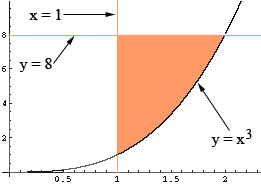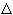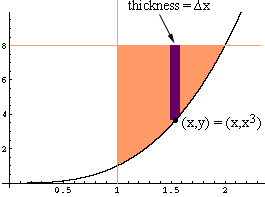I need to find out the answer for the below math question. Find the exact volume in cubic units generated by rotating a region, R, around the y-axis, given that R is the region bounded by the curve y = x3 and the lines x = 1 and y = 8. Your help would be appreciated. Thank you. Colleen for secondary school parent of student Hi Colleen, I plotted a sketch of y = x3, y = 8 and x = 1 and shaded the region R.The region shaded orange is the region you need to revolve around the y-axis. Either the disk or shell method will work but I think in this situation the cylindrical shell method results in an easier integral. To use the cylindrical shell method take a vertical slice through the region R of thicknessx and revolve this slice around the y-axis.Is this enough help? Let us know. Penny